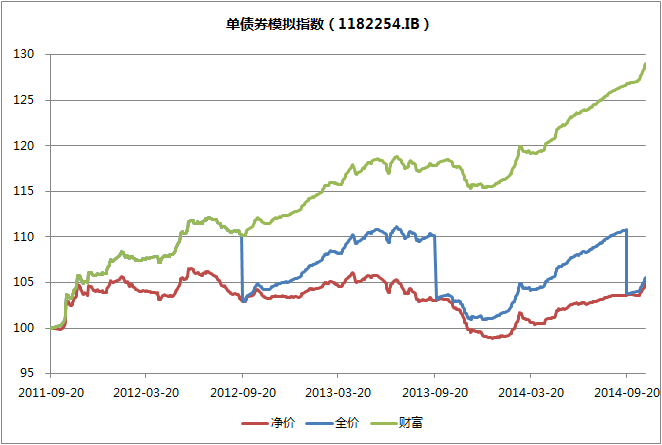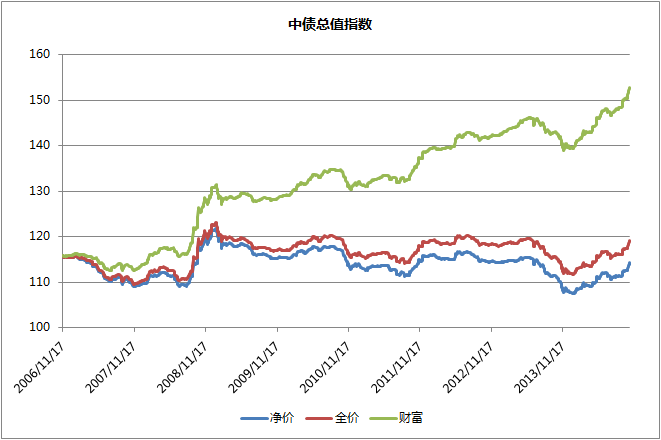# 债券的净价、全价和财富指数的区别和用法

$$I^F_T = I^F_{T-1}\sum_i W^F_{i,T-1}\frac{P^F_{i,T}}{P^F_{i,T-1}}$$

$$I^N_T = I^N_{T-1}\sum_i W^N_{i,T-1}\frac{P^N_{i,T}}{P^N_{i,T-1}}$$

$$I^{TR}_T = I^{TR}_{T-1}\sum_i W^{TR}_{i,T-1}\frac{P^F_{i,T}+PIN_{i,T}+INT_{i,T}}{P^F_{i,T-1}}$$• 净价指数用来衡量债券市场的涨跌，它和收益率的变动相反。
• 财富指数用来做绩效比较、业绩基准。

1. 从公式上看，全价指数和净价指数没有考虑本金的提前支付。如果有本金支付，指数会异常下跌。我不确定这只是计算公式的疏忽还是真实处理就是如此。
2. 中债-新综合指数是中债最新发布的指数，其中再投资的处理从之前的立即再投资改成按日累计到月末再集中再投资。我不确定这种处理方法的意图何在，除了增加计算的复杂性外。

Q. E. D.

IT » css, 打印